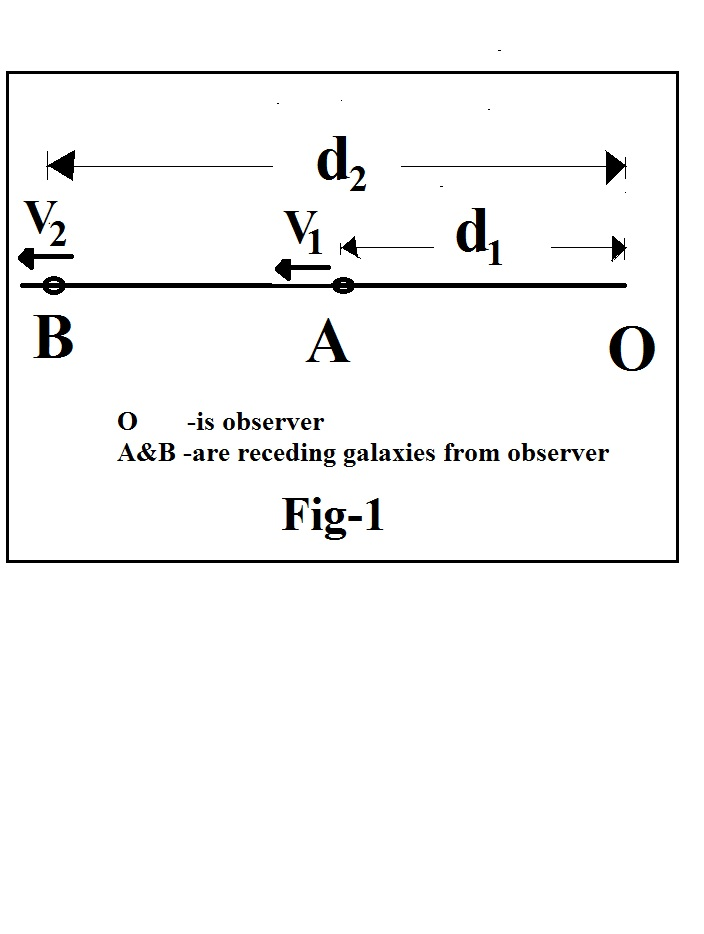Preprint Article Version 1 Preserved in Portico This version is not peer-reviewed

# Theoretical Value of Hubble’s constant is a Salient Feature of Experimental Results- New Insight in to Origin of Universe

Version 1 : Received: 18 September 2020 / Approved: 19 September 2020 / Online: 19 September 2020 (08:31:12 CEST)

How to cite: Kodukula, S.P. Theoretical Value of Hubble’s constant is a Salient Feature of Experimental Results- New Insight in to Origin of Universe. Preprints 2020, 2020090452 (doi: 10.20944/preprints202009.0452.v1). Kodukula, S.P. Theoretical Value of Hubble’s constant is a Salient Feature of Experimental Results- New Insight in to Origin of Universe. Preprints 2020, 2020090452 (doi: 10.20944/preprints202009.0452.v1).

## Abstract

Specifically this work is based on the concept of ‘dark energy’ as a phenomenal effect of expansion through which a theoretical value of Hubble’s constant has been introduced. It is a constant that is based on a postulate that the square root of ratio of the Siva’s constant ‘K’ and Hubble’s constant ‘H’ is exactly equal to the diameter of the neutral hydrogen atom. That is the, exact theoretical value of Hubble’s constant. This is always constant .However, space-time conversions and the changes in the velocity of light will affect the distance and velocity in Hubble’s equation. Deviation factors in velocity, distance and the Hubble’s constant have been calculated separately which are affected by change of velocity of light with expansion of space time. The observations are related to red shift. So the change factor in terms of Hubble’s constant has been calculated with respect to red shift. Thus a factor which affects the theoretical Hubble’s constant has been calculated to find the exact value of Hubble’s constant that satisfies the experimental results. The present theoretical value of Hubble’s constant has been calculated. The experimental results by plank, ACT (Atacama Cosmology Telescope), WMAP (Wilkinson Microwave Anisotropy Probe) etc. experiments are at par with the theoretical result. Thus the ontology of space time introduced in this theoretical work supposed to be correct and leads to a new theory of origin of universe for which a brief description has been provided.## Subject Areas

Hubble’s constant ‘H’; space time; velocity of light; parsec; expanding universe; Siva’s constant ‘K’

Views 0